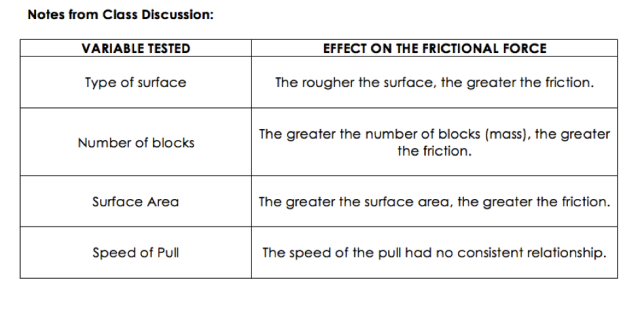# Friction Lab, Day 2

1 teachers like this lesson
Print Lesson

## Objective

Students will be able to define friction and describe the major factors that influence the magnitude of the force of friction acting on an object sliding on a surface.

#### Big Idea

Students apply conclusions they draw about the factors that affect friction to new situations.

## Friction Lab Discussion

30 minutes

The goal of this lesson is for students to gain a better understanding of what friction is and how it affects objects in motion. Students will begin by discussing the results from their lab of how different factors affect the friction on an object (SP8). Additionally, students will use the model of Newton's second law that they have created (HS-PS2-1), where acceleration = Fnet/mass, to analyze different problems where they must consider how friction affects the net force and the acceleration.

To start this lesson, I ask students to take out their Friction Lab and spend about 5 minutes reviewing the information they collected as a group during the lab in the previous lesson. If they haven't yet finished the analysis questions, I ask them to complete those. When students have refreshed their memories, I ask them to explain what they did in the lab and the variables they investigated. To do this, we first list the variables, as shown below.After we list the variables investigated, I ask students to compare their results with another table to see if they have similar data. Students share out their results to the class and why they think the variable does or does not affect friction. To do this, I call on volunteers that would like to explain one factor they found to affect friction. We go through each variable until the discussion notes are completed, as shown below.At the end of the discussion, students identify that the factors that influence the amount friction on an object are: the mass of the object, the surface area of the object and the surface type.

## Air Resistance Application WS

35 minutes

After students have determined the different factors that influence friction, I tell students that air resistance is a type of friction and that they will be completing a the Friction and Air Resistance Concept Development Practice Page (WS 6-1), from the Conceptual Physics textbook by Hewitt, where they will be analyzing what happens in a few situations where there is friction or air resistance. The purpose of doing this worksheet is for students to find how friction acts in different cases to reduce the net force acting on an object, causing it to have a smaller acceleration. I ask them to work with the same partner that they worked with the lab on and I give them about 15 minutes to complete the worksheet. I tell them that they must show all work for any questions that require equations.

After students have finished the worksheet, I ask them to compare with another group to see if they agree on the answers. When everyone in the class has compared their answers, we review the worksheet as a class. Since they have had an opportunity to work with a partner and compare with another group, I take this opportunity to make sure all of my students participate by calling randomly on a student to provide an answer. Since there are so many questions on the worksheet and because students have received feedback from their peers, this should be low-risk even for students who don't like to participate.

## Exit Slip

5 minutes

To end class, I want to see what students have learned about friction. I ask them to take out a notecard from the center bin and answer the two questions that I have written on the board:

1. Which direction does friction typically act in?

2. List two factors that influence friction and explain how you know.

I use this to help see if students have understood the friction typically acts in the opposite direction of the direction of motion or net force and to see what factors influence friction as seen in the student work here (Friction Exit Slip). I grade this and input it as a formative because by this point students should know enough about friction to answer these questions.